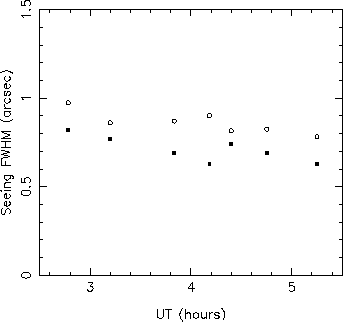# The Outer Scale of Turbulence

 Noll's analysis Noll's analysis for the variances of the Zernike mode coefficient fluctuations due to atmospheric seeing assumes that the outer scale of turbulence is effectively infinite. However, unless L0 is several orders of magnitude larger than the telescope diameter, there will be a measurable reduction in the strength of the lowest order modes relative to the case of infinite L0. We expect L0 to be of the order of the thickness of a typical turbulent layer, i.e. a few tens of metres. Hence a significant reduction in the strengths of the lowest order modes over their Noll values may be expected for the 4.2m WHT. Winker  (1991) and Jenkins  (1998) have extended Noll's analysis to give the variances of Zernike coefficients for the case of finite L0. The strength of the lowest order modes is substantially reduced, whereas higher orders are relatively unaffected. This permits an estimation of L0 for the JOSE data from the relative variances of the low and high order modes. The effect should be strongest for the first order Zernike modes, for example reducing the rms values of the Zernike tilts for the WHT by 50%  for L0 = 20m .  The true rms values cannot be deduced from the JOSE data, because  atmospherically induced tilts cannot be distinguished reliably from slow telescope tracking errors.  However, we have seen that the variances of tilt modes for the JOSE  data are in most cases smaller than their theoretical values for L0 = infinity (figure). The reduction of the rms values for second order terms (eg. defocus) term is much smaller, but is unaffected by telescope tracking errors, and hence provides an unbiased estimator for L0.  The mean value of L0 for the JOSE data, estimated from the ratio of the variances of the second order Zernike coefficients to those for higher orders, is 15 metres. For the finite outer scale case, the FWHM is no longer given by  0.98lambda/r0. The analysis of Wilson and Jenkins  (1996) yields the expected PSF for a given combination of  r0, L0 and telescope diameter. For L0 = 15m the median FWHM intrinsic seeing value for the WHT is reduced from 0.69 to 0.55 arcseconds at a wavelength of 700nm. At shorter wavelengths the effects of the finite outer scale are less significant, and we expect a FWHM closer to the DIMM prediction. The figure below demonstrates that FWHM values smaller than the DIMM prediction can be achieved in practice. The FWHM for CCD images of stars recorded at the Auxiliary port focus of the WHT on October 25, 1997 are plotted with the DIMM seeing predictions for the same night. The DIMM seeing values were averaged over the duration of each CCD exposure. The CCD images were recorded  at a wavelength of 657nm, with good spatial sampling (0.1 arcsec/pixel), and care was taken to maintain optimum focus of the telescope throughout. The mean ratio of the measured FWHM to the DIMM predictions is consistent with an outer scale length of approximately 20 metres.Measurements of the FWHM of stellar images at the WHT Auxiliary focus for October 25, 1997 (filled squares), with contemporaneous DIMM seeing estimates (open circles).   References: Winker, D.M., 1991, J.Opt.Soc.Am., 8, 1568. Jenkins, C.R., 1998, MNRAS, 268, 39. Wilson, R.W., & Jenkins, C.R., 1996, MNRAS, 268, 39.